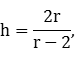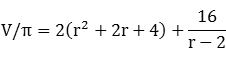Equable Cylinder

EQUABLE CYLINDER

Balmoral Software

Solutions: 5

For a right circular cylinder with radius r and height h, we have
V = π r2h

S = 2π rh + 2π r2

By convention, the volume and the circular base area are integer multiples of π, so r2h and r2 are integers and therefore h is rational. The lateral surface area is also an integer multiple of π, so 2rh is an integer and therefore r is also rational. Since r2 is an integer and r is rational, it follows that r is an integer.

By equability,

r2h = 2rh + 2r2

 (r - 2)(h - 2) = 4 

A right cylinder is a right prism with a circular base, so h > 2 as is established elsewhere. It follows that r > 2 in any equable solution. We then haveandis an integer. Then r - 2 divides 16. Therefore, r ∈ {3,4,6,10,18} and we have the following five equable solutions:
rhr22rhV/π=S/π
3693654
44163264
633636108
105/210050250
189/432481729
It follows from  that rh = 2(r + h), so every equable cylinder is created from a solid of rotation of an equable r x h rectangle rotated around any of its sides (although it may not have purely-integer dimensions). Three of the five equable cylinders have integer dimensions.